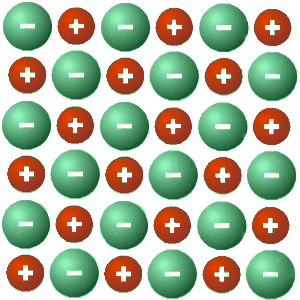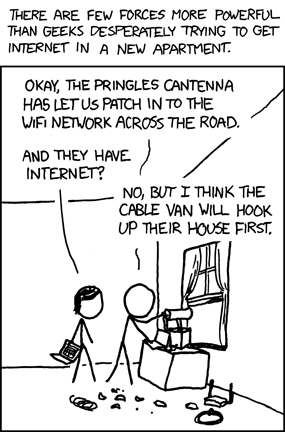# Monthly Archives: October 2016

## Halloween Humor…

Click the image for similar cartoons…Thanks to Judy Nguyen who shared these with me!

## Bohr-van Leeuwen Theorem and Micro/Macro Disconnect

A couple weeks ago, I wrote a post about the Gibbs paradox and how it represented a case where, if particle indistinguishability was not taken into account, led to some bizarre consequences on the macroscopic scale. In particular, it suggested that entropy should increase when partitioning a monatomic gas into two volumes. This paradox therefore contained within it the seeds of quantum mechanics (through particle indistinguishability), unbeknownst to Gibbs and his contemporaries.

Another historic case where a logical disconnect between the micro- and macroscale arose was in the context of the Bohr-van Leeuwen theorem. Colloquially, the theorem says that magnetism of any form (ferro-, dia-, paramagnetism, etc.) cannot exist within the realm of classical mechanics in equilibrium. It is quite easy to prove actually, so I’ll quickly sketch the main ideas. Firstly, the Hamiltonian with any electromagnetic field can be written in the form:$H = \sum_i \frac{1}{2m_i}(\textbf{p}_i - e\textbf{A}_i)^2 + U_i(\textbf{r}_i)$

Now, because the classical partition function is of the form:$Z \propto \int_{-\infty}^\infty d^3\textbf{r}_1...d^3\textbf{r}_N\int_{-\infty}^\infty d^3\textbf{p}_1...d^3\textbf{p}_N e^{-\beta\sum_i \frac{1}{2m_i}(\textbf{p}_i - e\textbf{A}_i)^2 + U_i(\textbf{r}_i)}$

we can just make the substitution:$\textbf{p}'_i = \textbf{p}_i - e\textbf{A}_i$

without having to change the limits of the integral. Therefore, with this substitution, the partition function ends up looking like one without the presence of the vector potential (i.e. the partition function is independent of the vector potential and therefore cannot exhibit any magnetism!).

This theorem suggests, like in the Gibbs paradox case, that there is a logical inconsistency when one tries to apply macroscale physics (classical mechanics) to the microscale and attempts to build up from there (by applying statistical mechanics). The impressive thing about this kind of reasoning is that it requires little experimental input but nonetheless exhibits far-reaching consequences regarding a prevailing paradigm (in this case, classical mechanics).

Since the quantum mechanical revolution, it seems like we have the opposite problem, however. Quantum mechanics resolves both the Gibbs paradox and the Bohr-van Leeuwen theorem, but presents us with issues when we try to apply the microscale ideas to the macroscale!

What I mean is that while quantum mechanics is the rule of law on the microscale, we arrive at problems like the Schrodinger cat when we try to apply such reasoning on the macroscale. Furthermore, Bell’s theorem seems to disappear when we look at the world on the macroscale. One wonders whether such ideas, similar to the Gibbs paradox and the Bohr-van Leeuwen theorem, are subtle precursors suggesting where the limits of quantum mechanics may actually lie.

## LST Relation – The Physical Picture

In 1941, Lydanne, Sachs and Teller wrote a paper entitled “On the Polar Vibrations of Alkali Halides”, where they derived a result now known as the Lydanne-Sachs-Teller (LST) relation. It has wide applicability for polar insulators. I reproduce the relation below:$\frac{\omega_{LO}^2}{\omega_{TO}^2} = \frac{\epsilon(o)}{\epsilon(\infty)}$

In the equation above,$\omega_{LO}$ and$\omega_{TO}$ refer to the frequencies of the longitudinal and transverse optical phonons respectively.$\epsilon(0)$ and$\epsilon(\infty)$ refer to the static and high frequency (above the phonon frequencies, but below any electronic energy scale) dielectric constants. All these quantities are understood to be the values in the long-wavelength limit (i.e.$q \approx 0$).

The beautiful thing about the LST result is that it is independent of any microscopic description, which is quite unusual in solid-state physics. Therefore, the result can be derived from classical electrodynamics, without resorting to any quantum mechanics. It is an interesting question as to whether or not quantum mechanics plays a role in the long-wavelength optical response in general.

Regardless, it turns out that all quantities in the LST relation are experimentally accessible! I find this relation quite remarkable and deep. Not only that, the agreement with experiment in many polar semiconductors is excellent. Take a look at the table below to get an idea of how well this relation holds for a few materials (reproduced from Mark Fox’s textbook Optical Properties of Solids):I have found textbook derivations don’t give a good intuition of why this relation holds, so here is my attempt to rectify this situation. First, let me state an important assumption that goes into the LST relation:

The phonons are assumed to be in the harmonic limit (i.e. no phonon anharmonicity) and as a result, the dielectric constant has the following form:$\epsilon(\omega) = \epsilon(\infty) + \frac{C}{\omega_{TO}^2-\omega^2}$

where$C$ is a constant. This form of the dielectric constant can be arrived at using either classical electrodynamics or quantum mechanics (see e.g. Ashcroft and Mermin, Kittel or Ziman).

Now, with this result under our belts, it turns out that it is quite simple to understand why the LST relation holds. In a simple polar semiconductor, we have two atoms per unit cell that are oppositely charged like so:Therefore, for the longitudinal optical phonon we have an extra polarization effect due to the long-range nature of the Coulomb interaction. This extra polarization results in an extra restoring force (in addition to the springy restoring force between the ions), yielding a higher longitudinal phonon frequency compared to the transverse optical phonon. I have discussed this a little more extensively in a previous post. This extra restoring force (which is only present for the longitudinal oscillation) is pictured below:The longitudinal optical phonon is at a higher energy because of the extra Coulombic polarization effect

More precisely, we can write the following when including this extra restoring force:$\omega_{LO}^2 = \omega_{TO}^2 + \frac{C}{\epsilon(\infty)}$

There is an$\epsilon(\infty)$ in the formula above because this polarization will necessarily be screened by higher energy (electronic) processes. Dividing both sides by$\omega_{TO}^2$, we can write the above equation suggestively as:$\frac{\omega_{LO}^2}{\omega_{TO}^2} = \frac{\epsilon(\infty)+C/\omega_{TO}^2}{\epsilon(\infty)}$

Looking at the equation for the dielectric constant from earlier, this is precisely the LST relation! In effect, the same extra restoring due to the long-range Coulomb interaction leads to the extra screening in the static limit, yielding, in my mind, a delightful little result.

Using the LST relation, we can deduce a property of ferroelectric materials. Namely, we know that at the transition temperature between the normal state and a ferroelectric ground state, the static dielectric constant,$\epsilon(0)$, diverges. Therefore, we can surmise from the LST relation that a zone center transverse optical phonon must go to zero energy (soften) at the transition temperature (see here for PbTiO3). This is a totally non-trivial consequence of the LST relation, demonstrating again its far-reaching utility.

Did I mention that I think this result is pretty excellent?

I’d like to acknowledge Zhanybek Alpichshev for enlightening some aspects regarding this topic.

## Too Close to Home

I haven’t been blogging much recently because I just moved from Chicago to Boston. Also, I don’t currently have access to internet in my new apartment. As always, there’s an XKCD comic to capture this scenario:Hopefully, I’ll be back and posting more often soon!

## An Interesting Research Avenue, an Update, and a Joke

An Interesting Research Avenue: A couple months ago, Stephane Mangin of the Insitut Jean Lamour gave a talk on all-optical helicity-dependent magnetic switching (what a mouthful!) at Argonne, which was fascinating. I was reminded of the talk yesterday when a review article on the topic appeared on the arXiv. The basic phenomenon is that in certain materials, one is able to send in a femtosecond laser pulse onto a magnetic material and switch the direction of magnetization using circularly polarized light. This effect is reversible (in the sense that circularly polarized light in the opposite direction will result in a magnetization in the opposite direction) and is reproducible. During the talk, Mangin was able to show us some remarkable videos of the phenomenon, which unfortunately, I wasn’t able to find online.

The initial study that sparked a lot of this work was this paper by Beaurepaire et al., which showed ultrafast demagnetization in nickel films in 1996, a whole 20 years ago! The more recent study that triggered most of the current work was this paper by Stanciu et al. in which it was shown that the magnetization direction could be switched with a circularly polarized 40-femtosecond laser pulse on ferromagnetic film alloys of GdFeCo. For a while, it was thought that this effect was specific to the GdFeCo material class, but it has since been shown that all-optical helicity-dependent magnetic switching is actually a more general phenomenon and has been observed now in many materials (see this paper by Mangin and co-workers for example). It will be interesting to see how this research plays out with respect to the magnetic storage industry. The ability to read and write on the femtosecond to picosecond timescale is definitely something to watch out for.

Update: After my post on the Gibbs paradox last week, a few readers pointed out that there exists some controversy over the textbook explanation that I presented. I am grateful that they provided links to some articles discussing the subtleties involved in the paradox. Although one commenter suggested Appendix D of E. Atlee Jackson’s textbook, I was not able to get a hold of this. It looks like a promising textbook, so I may end up just buying it, however!

The links that I found helpful about the Gibbs paradox were Jaynes’ article (pdf!) and this article by R. Swendsen. In particular, I found Jaynes’ discussion of Whifnium and Whoofnium interesting in the role that ignorance and knowledge plays our ability to extract work from a partitioned gases. Swendsen’s tries to redefine entropy classically (what he calls Boltzmann’s definition of entropy), which I have to think about a little more. But at the moment, I don’t think I buy his argument that this resolves the Gibbs paradox completely.

A Joke:

Q: What did Mrs. Cow say to Mr. Cow?

A: Hubby, could you please mooo the lawn?

Q: What did Mr. Cow say back to Mrs. Cow?

A: But, sweetheart, then what am I going to eat?